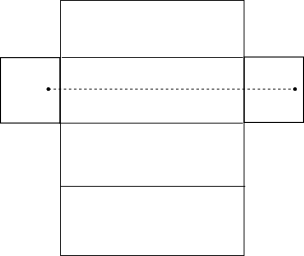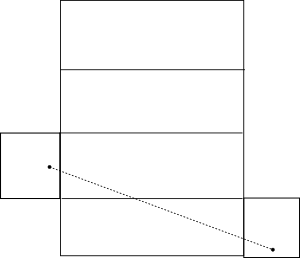#The Spider and the Fly

Math Lair Home > Puzzles & Problems > The Spider and the Fly

Henry E. Dudeney described the following puzzle in the Weekly Dispatch of June 14, 1903:

Inside a rectangular room, measuring 30 feet in length and 12 feet in width and height, a spider is at a point on the middle of one of the end walls, 1 foot from the ceiling, as at A; and a fly is on the opposite wall, 1 foot from the floor in the centre, as shown at B. What is the shortest distance that the spider must crawl in order to reach the fly, which remains stationary? Of course the spider never drops or uses its web, but crawls fairly.

Solution:

Let's start by considering a simpler problem. Imagine that the spider and the fly are on adjacent walls, and say that the spider wants to walk along the walls of the room to the location of the fly, along the shortest possible path. What would that path be? We could find the shortest path by unfolding the two walls, and then drawing a straight line between the spider and the fly.

The way to solve the original problem is analogous to this easier problem. We can treat the room as a rectangular prism, unfold it, and draw a line between the spider and the fly.

It's slightly more complicated this time, as we can unfold the prism in many ways. Since each end wall can be attached to one of four walls (ceiling, floor, long wall #1 and long wall #2), there are sixteen different ways to unfold the walls. However, because of the symmetry in the problem, we need only consider four of them. We can then use the Pythagorean theorem to figure out the shortest distance. Note that I've assumed below that the room is oriented so that the spider is on the east wall and the fly on the west wall just for the sake of simplifying the descriptions; of course, it doesn't matter how the room is oriented.
DescriptionDiagramDistance
End walls hinged to floor1 + 30 + 11 = 42
End walls hinged to north wall(6 + 30 + 6)² + 10² ≈ 43.2
West wall hinged to floor, east wall hinged to south wall(1 + 30 + 6)² + (6 + 11)² ≈ 40.7
West wall hinged to floor, east wall hinged to ceiling(1 + 30 + 1)² + (6 + 12 + 6)² = 40

The shortest result is the last one in the table. Perhaps this is a slightly unintuitive result, because the shortest path ends up passing through five of the six of the walls of the room, but that is the shortest solution.

View other puzzles and problems at the Math Lair.

Sources used (see bibliography page for titles corresponding to numbers): 7, 49.